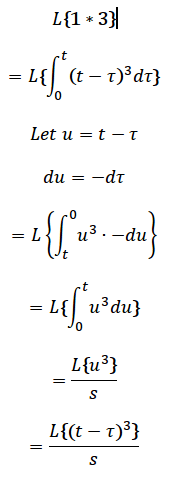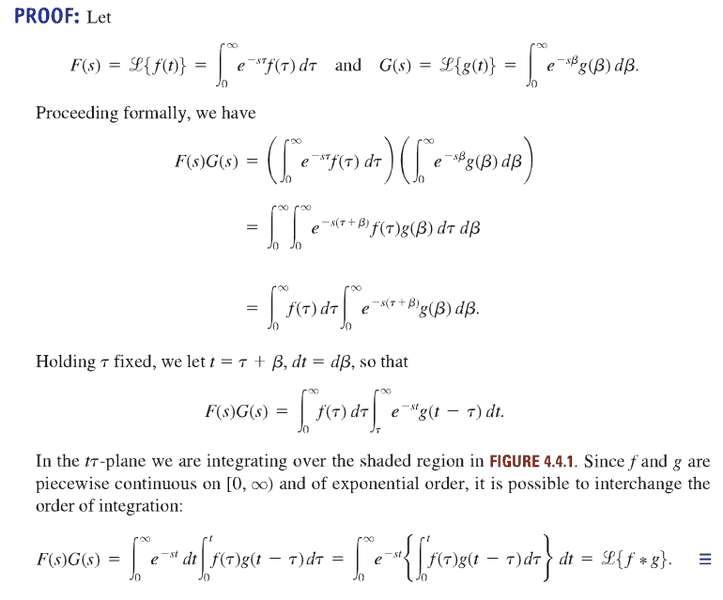# Don't understand this application of the transform of an integral

• Turion

## Homework Statement

$$L\{ 1*{ t }^{ 3 }\}$$

## Homework Equations

$$L\{ \int _{ 0 }^{ t }{ f(\tau )d\tau } \} =\frac { L\{ f(t)\} }{ s }$$

## The Attempt at a Solution

$$L\{ 1*{ t }^{ 3 }\} \\ =L\{ \int _{ 0 }^{ t }{ { (t-\tau ) }^{ 3 }d\tau } \} \\ =\frac { L\{ { t }^{ 3 }\} }{ s } \\ =\frac { 6 }{ { s }^{ 5 } }$$

The function in the integral is a function of t and tau so how was the identity under "relevant equations" applied?

Yes, you have ##\int_0^tf(\tau,t)\,d\tau## in the integral instead of ##\int_0^tf(\tau)\,d\tau## and the formula for integral doesn't work. This is convolution so:

##L\{1*t^3\}=L\{1\}\cdot L\{t^3\}##

## Homework Statement

$$L\{ 1*{ t }^{ 3 }\}$$

## Homework Equations

$$L\{ \int _{ 0 }^{ t }{ f(\tau )d\tau } \} =\frac { L\{ f(t)\} }{ s }$$

## The Attempt at a Solution

$$L\{ 1*{ t }^{ 3 }\} \\ =L\{ \int _{ 0 }^{ t }{ { (t-\tau ) }^{ 3 }d\tau } \} \\ =\frac { L\{ { t }^{ 3 }\} }{ s } \\ =\frac { 6 }{ { s }^{ 5 } }$$

The function in the integral is a function of t and tau so how was the identity under "relevant equations" applied?
Use the substitution ##u=t-\tau## on the integral to convert it to a more familiar form.

@szynkasz: Thanks. I solved it.

Use the substitution ##u=t-\tau## on the integral to convert it to a more familiar form.Any suggestions?

Remember that ##u## is a dummy variable.

Remember that ##u## is a dummy variable.

Yeah, I substituted it back in the last step so there is no more u. I'm not sure how that would help me.

I don't think you understood my point. In the integral ##\int_0^t u^3\,du##, it doesn't matter what letter we use for the variable of integration. It's a dummy variable.

http://mathworld.wolfram.com/DummyVariable.html

If you evaluated the integral, what variable is it a function of?

I don't think you understood my point. In the integral ##\int_0^t u^3\,du##, it doesn't matter what letter we use for the variable of integration. It's a dummy variable.

http://mathworld.wolfram.com/DummyVariable.html

If you evaluated the integral, what variable is it a function of?

It's a function of u which is the same thing as saying it's a function of t - tau.

Really? Did you actually calculate ##\int_0^t u^3\,du##?

Really? Did you actually calculate ##\int_0^t u^3\,du##?

No, I didn't. The textbook says to apply the identity under "2. Homework Equations " first.

You wrote
$$L\{ \int _{ 0 }^{ t }{ f(\tau )d\tau } \} =\frac { L\{ f(t)\} }{ s }$$
What variable is ##f## a function of on the left-hand side? What variable is ##f## a function of on the right-hand side? Are they the same? Why not?

You wrote
What variable is ##f## a function of on the left-hand side? What variable is ##f## a function of on the right-hand side? Are they the same? Why not?

On the left hand side, f is a function of tau. On the right hand side, f is a function of t. They are not the same. I'm not sure why they're not the same but I can dig up the proof for it I guess. I think they're not the same because we're doing a transformation.

Last edited:
I found the proof:Honestly, that proof (as most proofs) went right over my head.